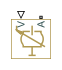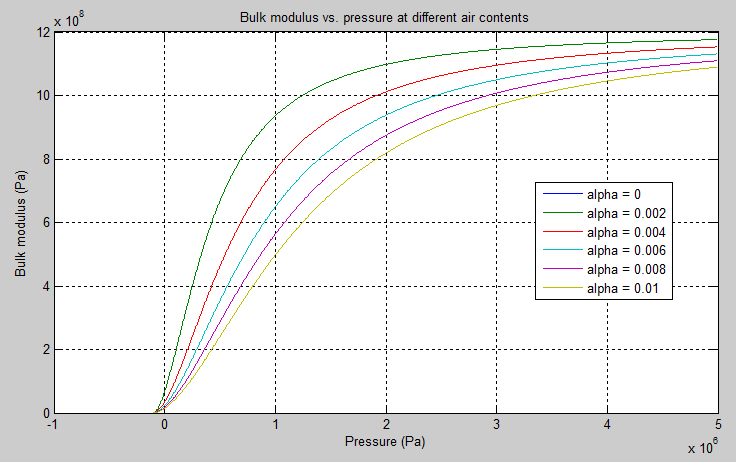# Variable Hydraulic Chamber

(To be removed) Hydraulic capacity of variable volume with compressible fluid

The Hydraulic library will be removed in a future release. Use the Isothermal Liquid library instead. (since R2020a)

## Library

Hydraulic Elements

•## Description

The Variable Hydraulic Chamber block models fluid compressibility in variable volume chambers. The fluid is considered to be a mixture of liquid and a small amount of entrained, nondissolved gas. Use this block together with the Translational Hydro-Mechanical Converter block.

Note

The Variable Hydraulic Chamber block takes into account only the flow rate caused by fluid compressibility. The fluid volume consumed to create piston velocity is accounted for in the Translational Hydro-Mechanical Converter block.

The chamber is simulated according to the following equations (see [1, 2]):

`$q=\frac{{V}_{0}+V}{E}·\frac{dp}{dt}$`
`$E={E}_{l}\frac{1+\alpha {\left(\frac{{p}_{a}}{{p}_{a}+p}\right)}^{1/n}}{1+\alpha \frac{{p}_{a}^{1/n}}{n·{\left({p}_{a}+p\right)}^{\frac{n+1}{n}}}{E}_{l}}$`

where

 q Flow rate due to fluid compressibility V0 Initial volume of fluid in the chamber V Chamber volume change, provided through port V E Fluid bulk modulus El Pure liquid bulk modulus p Gauge pressure of fluid in the chamber pα Atmospheric pressure α Relative gas content at atmospheric pressure, α = VG/VL VG Gas volume at atmospheric pressure VL Volume of liquid n Gas-specific heat ratio

The main objective of representing fluid as a mixture of liquid and gas is to introduce an approximate model of cavitation, which takes place in a chamber if pressure drops below fluid vapor saturation level. As it is seen in the graph below, the bulk modulus of a mixture decreases as the gauge pressure approaches zero, thus considerably slowing down further pressure change. At gauge pressures far above zero, a small amount of undissolved gas has practically no effect on the system behavior.For information on how to reproduce this graph, see Constant Volume Hydraulic Chamber.

Cavitation is an inherently thermodynamic process, requiring consideration of multiple-phase fluids, heat transfers, etc., and as such cannot be accurately simulated with Simscape™ software. But the simplified version implemented in the block is good enough to signal if pressure falls below dangerous level, and to prevent computation failure that normally occurs at negative pressures.

If pressure falls below absolute vacuum (–101325 Pa), the simulation stops and an error message is displayed.

Port A is a hydraulic conserving port associated with the chamber inlet. Port V is a physical signal port that provides the chamber volume variation.

The block positive direction is from port A to the reference point. This means that the flow rate is positive if it flows into the chamber.

### Variables

Use the Variables tab to set the priority and initial target values for the block variables prior to simulation. For more information, see Set Priority and Initial Target for Block Variables.

## Basic Assumptions and Limitations

• Fluid density remains constant.

• Chamber volume cannot be less than the dead volume.

• Fluid fills the entire chamber volume.

## Parameters

Minimal volume of fluid in the chamber. The default value is `1e-4` m^3.

Specific heat ratio

Gas-specific heat ratio. The default value is `1.4`.

## Global Parameters

Parameters determined by the type of working fluid:

• Fluid density

• Fluid kinematic viscosity

Use the Hydraulic Fluid (Simscape Fluids) block or the Custom Hydraulic Fluid block to specify the fluid properties.

## Ports

The block has the following ports:

`A`

Hydraulic conserving port associated with the chamber inlet.

`V`

Physical signal port that provides the chamber volume variation.

## References

 Manring, N.D., Hydraulic Control Systems, John Wiley & Sons, New York, 2005

 Meritt, H.E., Hydraulic Control Systems, John Wiley & Sons, New York, 1967

## Version History

Introduced in R2009b

expand all